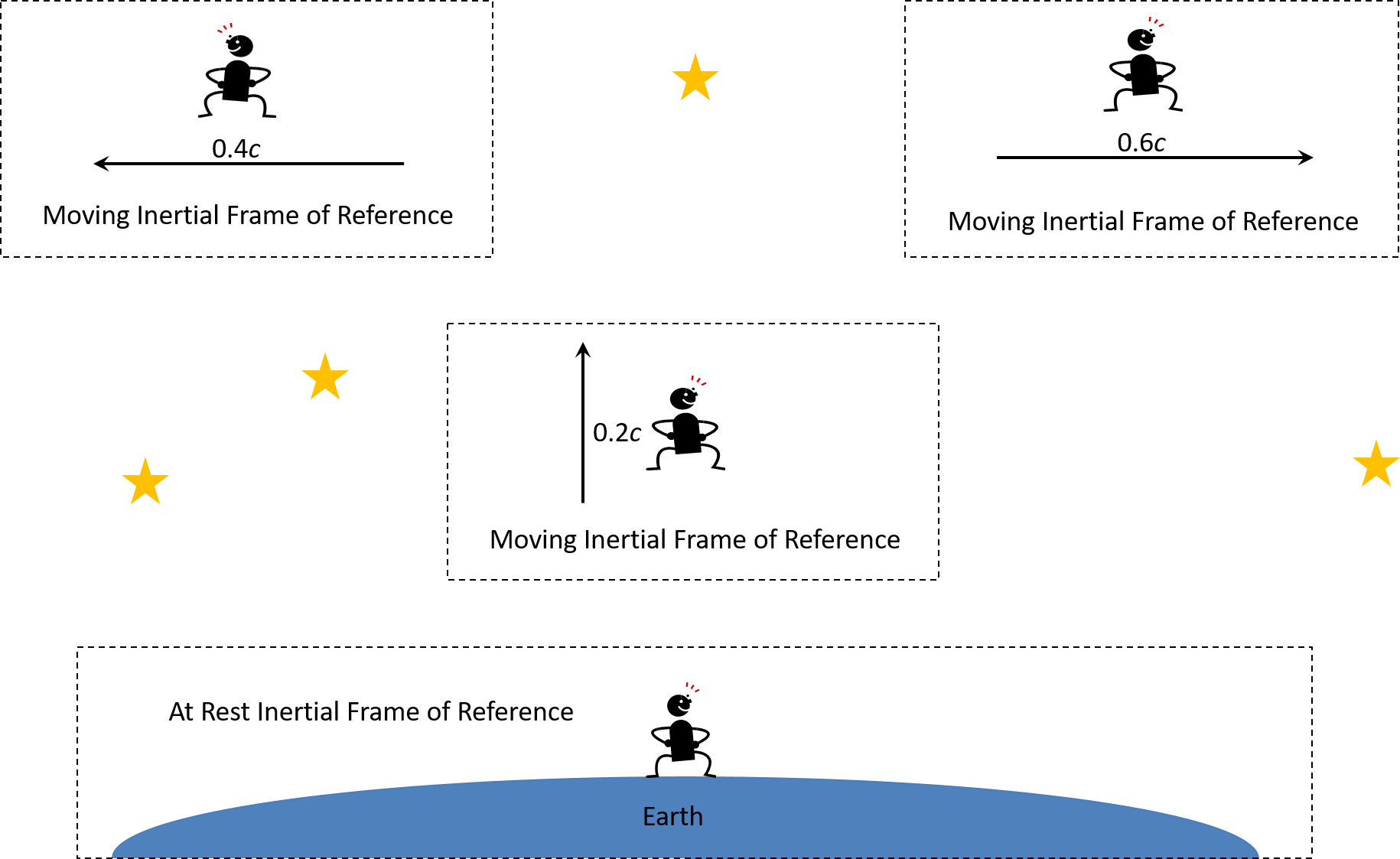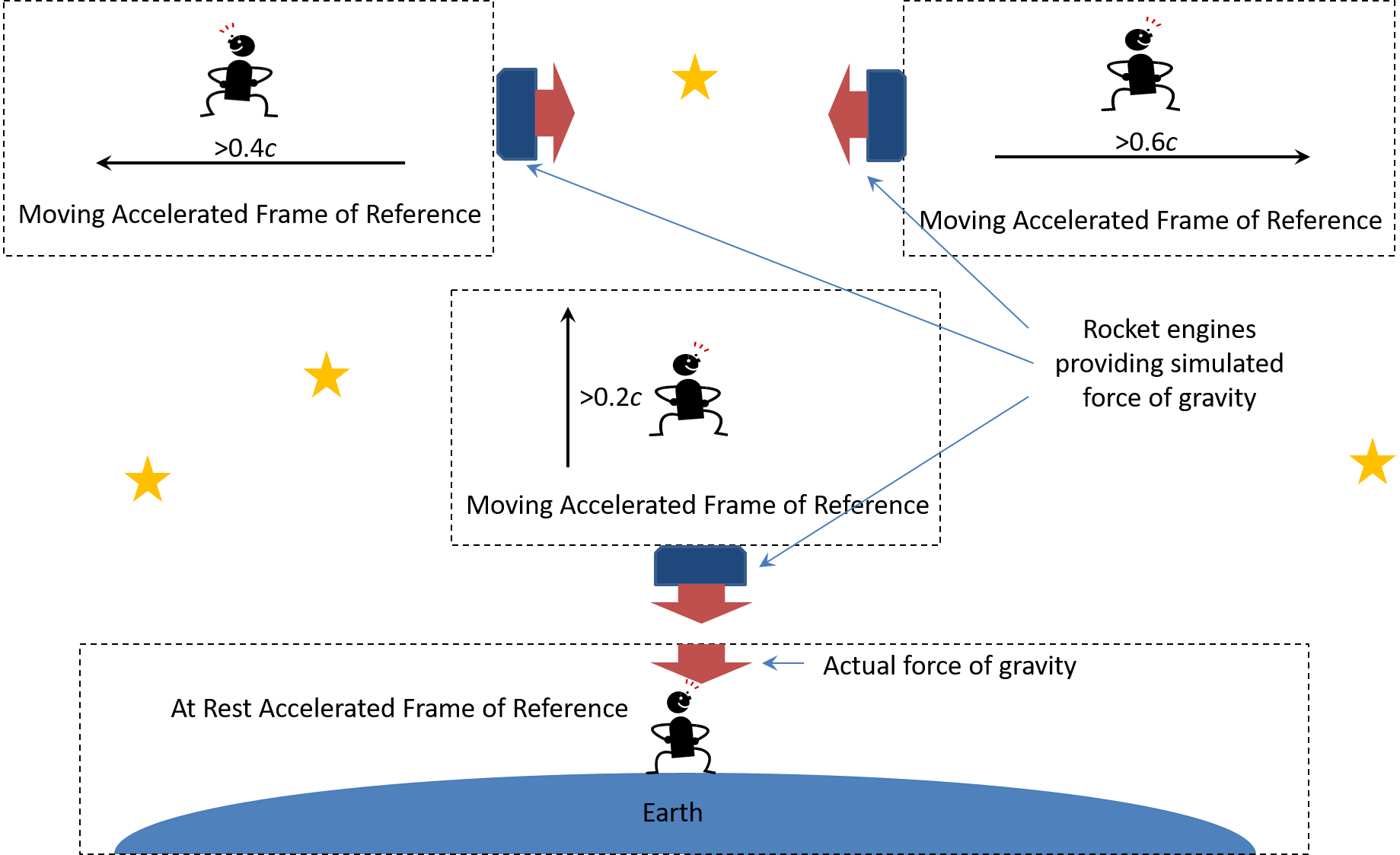# Principle of Relativity

The first assumption made by Albert Einstein was the Principle of Relativity. This principle states the laws of physics are the same in all frames of reference. In his Theory of Special Relativity, Einstein performed a mind experiment using Inertial Frames of Reference. In an Inertial Frame of Reference, an object is moving only under its own inertia (i.e. without any external force such as gravity). Inertia follows Newton’s First Law of Motion:

An object either remains at rest (At Rest Inertial Frame of Reference) or continues to move at a constant velocity (Moving Inertial Frame of Reference) unless acted upon by a force, which is not allowed in an Inertial Frames of Reference.

The following figure illustrates four Inertial Frames of Reference:The need for Inertial Frames of Reference comes from the fact that our universe has nothing that can be considered as standing still. As a result, one cannot calculate the speed and direction they are moving. At best, we can designate one frame of reference (e.g. an observer on Earth) to be considered as an At Rest Inertial Frame of Reference. All other frames of reference are Moving Inertial Frames of Reference and can make measurements relative to the one At Rest Inertial Frame of Reference. In the illustration above, we have three different Moving Inertial Frames of Reference moving in three different directions and speeds (fractions of the speed of light, c) relative to the observer on Earth.

The concept of Inertial Frames of Reference goes back to the time of Galileo in 1632 where he used the metaphor of Galileo’s Ship. Galileo’s Ship was another mind experiment based on a laboratory inside a ship with no windows to the outside world. The ship could be either standing still at a dock (i.e. At Rest Inertial Frame of Reference) or moving on smooth waters (i.e. Moving Inertial Frame of Reference). Inside the laboratory, all experiments would yield the exact same results so that the experimenter could not tell whether the ship was standing still or moving. All Inertial Frames of Reference must follow the same Newtonian laws of mechanics.

In Einstein’s General Theory of Relativity, Einstein added gravity to the Inertial Frames of Reference to create Accelerated Frames of Reference. The following figure illustrates our four previous Inertial Frames of Refence transformed into Accelerated Frames of Reference:In Accelerated Frames of Reference, there is again only one At Rest Accelerated Frame of Reference, but many Moving Accelerated Frames of Reference. The at rest frame of reference represents our observer on Earth under the influence of gravity. In all the moving frames of reference, gravity is simulated by rocket engines pushing the moving frames of reference with the same force as the gravity being experienced by the observer on Earth.

While many people think of an observer on Earth as being the at rest frame of reference, any of the moving frames of reference can be the at rest frame of reference. The previous at rest frame of reference now becomes a moving frame of reference relative to the new at rest frame of reference. All the other moving frames of reference are now measured relative to the new at rest frame of reference.

Regardless of which frame of reference is at rest and which frames of reference are moving, two properties must be observed:

1.       The laws of physics must be the same in all the frames of reference, and

2.       The experimenter cannot determine in which frame of reference the experiment is being performed.

The Romeo Effect series of books does not question the Principle of Relativity. It is considered to be a truth regarding our universe.